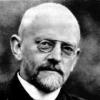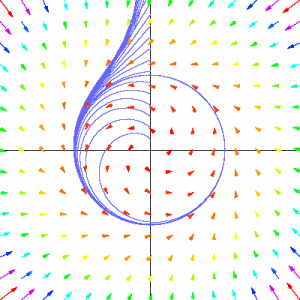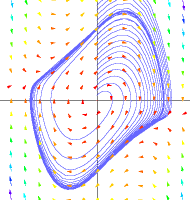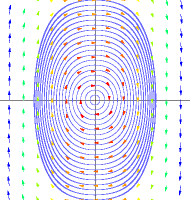Fall 2003

# Mathematics Math21b Fall 2003

## Linear Algebra and Differential Equations

Office: SciCtr 434
Email: knill@math.harvard.edu# Differential equations in the planeBecause a solution path of a differential equations can not cross itself, the dynamical in the place can not become too complicated. It is possible to have equilibrium points or limit cycles. Every orbit which does not start at an equilibrium point or on limit cicle either converges to one of these or it escapes to infinity. A famous problem of David Hilbert from 1900 asks how many limit cycles a polynomial differential equation can have.
 ``` d/dt x=ann xn yn+ ... + a11 xy + a10 x + a01 y + a0 d/dt y=bnn xn yn+ ... + a10 xy + b10 x + b01 y + b0 ```
in the plane can have.

 Are there only finitely many limit cycles? Is there a bound on their number which only depends on n?A special class of differential equations are Lienard systems
 ``` d2/dt2 x + F'(x) d/dt x + G'(x) = 0 ```
which is with y=d/dt x equivalent to
 ``` d/dt x = y d/dt y = -G'(x)-F'(x) y ```
or with y=d/dx +F, G'(x)=g equivalent to
 ``` d/dt x = y-F(x) d/dt y = -g(x) ```Example: van der Pool equation

 ``` d/dt x = y d/dt y = -x -(x2-1) y ```
Example: Duffing oscillator

 ``` d/dt x = y d/dt y = -x -x3 ```Last Wednesday, news broke that a student Elin Oxenhielm made progress on the Hilbert problem, claiming that a Lienard system with g(x)=x and F(x) a polynomial of degree 2k+1 has at most k limit cycles. The paper containing a sketch of a proof is here. Critics doubt that the proof will hold.

Back to the main page